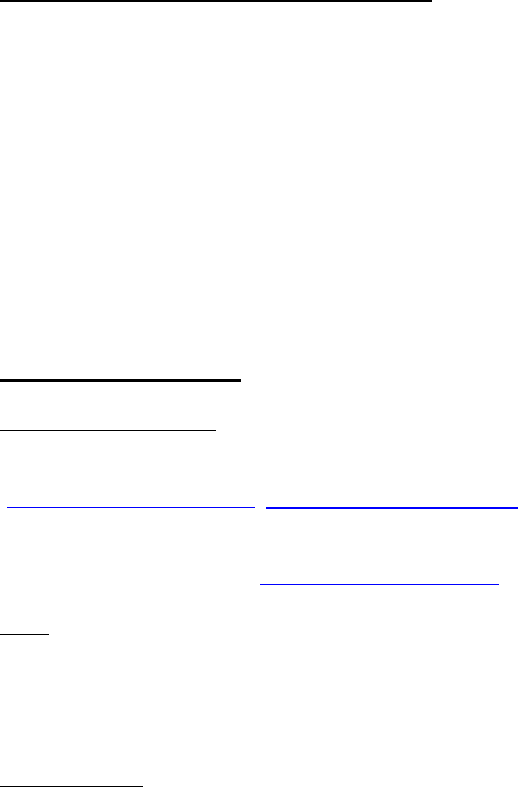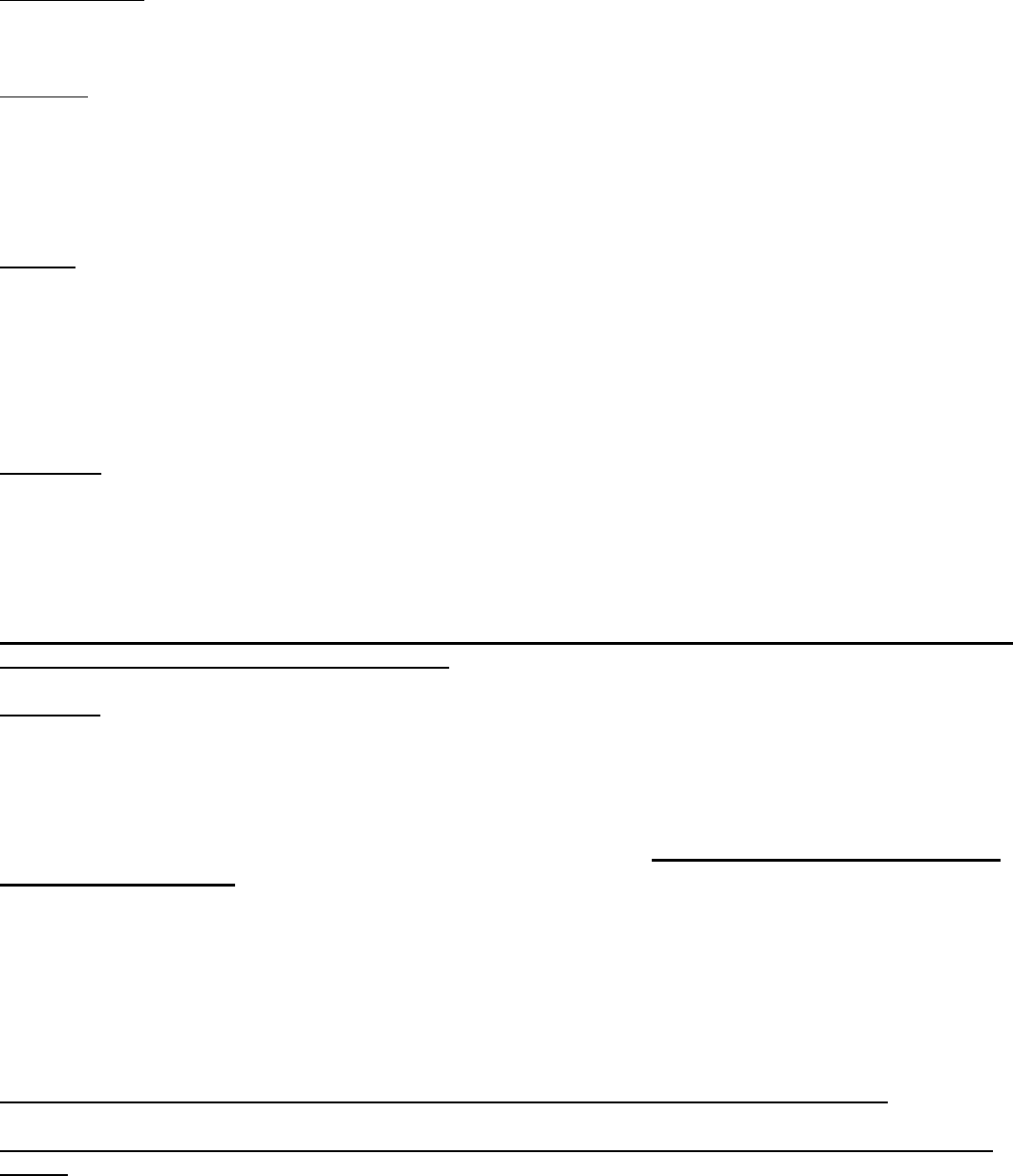# 01:960:285

Topics include descriptive statistics, probability, random variables, sampling distributions, principles of hypothesis testing, and one- and two-sample T-tests.## 24HR Notes for 01:960:285

Available 24 hours after each lecture

### Zhanyun Zhao

#### 01:960:285 Syllabus for Zhanyun Zhao — Fall 2018RUTGERS UNIVERSITY
DEPARTMENT OF STATISTICS
STAT 285:01– INTRODUCTORY STATISTICS FOR BUSINESS
FALL 2018
INSTRUCTOR
Zhanyun Zhao, Ph.D. Phone: 212-365-4088 e-mail: zhanyun.zhao@rutgers.edu
CLASS TIME
Tue / Fri 11:30 am 12:50 pm MI 100, CAC
TEXTBOOK
MCClave, Benson & Sincich, Statistics for Business and Economics. 13th Edition, Pearson 2016
(NOT 12th Edition, which has different homework problems)
PREREQUISITE
Pre-Calculus (Math 111 or equivalent)
COURSE OBJECTIVE
This course is designed to give the student an understanding of discrete and continuous random variables, the elements of
statistical inference, and an introduction to how these tools may be useful in one’s attempt to reach intelligent conclusions
in real-world settings. The focus is on the normal random variable, descriptive statistics, sampling distributions, and the
frameworks of estimation and hypothesis testing, particularly as they apply to inference for unknown population means
and proportions in the one- and two-sample settings.
LEARNING OBJECTIVES
Upon successful completion of the course, the student should be able to:
Graphically display (by hand and via Excel) qualitative and quantitative data via bar charts, pie graphs, dot plots,
stem-and-leaf displays, frequency and relative frequency histograms, and box-and-whisker plots
Compute and understand the data-descriptive usefulness of the statistics sample mode, median, mean, range,
variance and standard deviation, as well as the definitions of the parameters population mean, variance and
standard deviation
Compute quartiles and the interquartile range for a data set
Use the Empirical Rule and Chebyshev’s Theorem as applied to a data set
Understand the general concept of a continuous random variable and its probability density function, as well as
the idea (without calculus) of its mean, variance and standard deviation
Understand and solve problems involving the normal random variable
Use the normal approximation to the binomial random variable’s probability distribution
Understand the definitions of a simple random sample and the sampling distribution of a statistic
Compute the mean, variance and standard deviation of the sample mean
Understand and use the Central Limit TheoremUnderstand the general concept of a point and interval estimator
Compute and interpret a confidence interval for one population mean (large and small sample cases) and for one
population proportion (large sample case)
Compute the required sample size to estimate one population mean and one population proportion
Understand the general elements of a hypothesis testing problem, including the formulation of hypotheses, Type I
and Type II errors, a decision rule’s test statistic and rejection region, and the value of a test p.
Perform a test concerning one population’s mean (large and small sample cases) and one population’s proportion
(large sample case)
Compute a confidence interval for, and perform a test concerning, the difference of two population means (large
and small independent samples cases)
Compute a confidence interval for the difference, and perform a test of the equality, of two population proportions
(large independent samples case)
TEACHING AND LEARNING APPROACH
The instruction will consist of lectures, class discussions, lab assignments and homework problems. Each topic will be
introduced and example problems will be solved to demonstrate how the relevant method is applied.
Lecture notes will be posted in chapters on Sakai. I recommend that you have your lecture notes available each time you
come to class.
Homework problems will be assigned and collected periodically. You are expected to solve all homework problems by
the specified due date.
Lab assignments are group assignments that consist of hands-on activities for learning key statistical concepts and data
analysis techniques. We will have some time in class for Lab work, but the assignments will typically require additional
work outside of class.
TECHNICAL SUPPORT
MyMathLab/MyStatLab
MyMathLab/MyStatLab is delivered through Pearson’s MyLab and Mastering course management system.
(http://www.mymathlab.com, http://www.mystatlab.com) See attached flyers
You are NOT required to buy MyStatlab. If you choose to buy it, MyStatlab + loose leaf package at bookstore will give
Sakai
To facilitate class learning, please access and print course documents needed for class from the course management
system known as Sakai. Course documents are posted in folders under Resources. Also pay attentions to announcements
we have on Sakai.
Microsoft Excel
Microsoft Excel will be used for data analysis in this course. A basic working knowledge of Excel such as how to enter a
formula and working with cell references is assumed.Laptop computer
A laptop computer is optional in class to enhance your understanding of some key statistical concepts.
Calculator
A scientific calculator that has basic statistics and scientific functions is required. A graphing calculator is not necessary
but can be used to fulfill a calculator requirement. It is a good practice to bring your calculator to every class. Be sure to
bring your calculator to exams. Sharing calculators with your classmates during exams is strictly prohibited. Yet students
are not allowed to use statistical features on their calculators, if there are any.
EXAMS All exams are closed book, with one page of note sheet (front and/or back)
THERE WILL BE TWO MIDTERM EXAMS AND ONE COMPREHENSIVE FINAL EXAM.
YOU NEED TO HAVE A CALCULATOR FOR ALL EXAMS. YOU ARE NOT ALLOWED TO USE YOUR CELLPHONE
AS A CALCULATOR.
YOU HAVE TO SHOW YOUR WORK TO RECEIVE CREDIT FOR PROBLEMS ON EXAMS.
Final grades will be based on, homework assignments, lab assignments, exams and a comprehensive final exam using the
following weights:
Homework & quizzes 10%, Lab 10%, Midterms 20% each, and a Comprehensive Final Exam 40%
Check Registrar’s Schedule for Date & Time of the Final Exam. The final exam must be taken for this course.
If you have any questions concerning your grade, you can discuss with me within 7 days after the grade is posted.
Any arguments past 7 days will not be considered.
POLICIES
Assignments must be completed by the specified due date. In addition, exercises in the text similar to the ones assigned
should also be completed to assure sufficient practice and exposure to the material.
Show all work for assignments, quizzes and examinations to receive proper credit. If you do poorly on any quiz or exam,
you may have to submit class assignments at the discretion of the instructor. No exams or quizzes may be retaken in
Students who have legitimate scheduling conflicts with a exam must petition the instructor in person with written
verification and in advance to take a exam prior to the scheduled date.
If you miss an exam, it may not be made up unless official documentation/verification is given by an independent third
party and only for the reason of a major illness or major emergency. (Letters from guardians are not acceptable.) The
instructor should also be notified in advance that the exam will be missed. The exam must be made up within one week.
Academic dishonesty will not be tolerated. You must work on your own during quizzes and exams.
We will have a universal rule for letter grade for everybody, no special exception to bump up grade for specific
reasonsTENTATIVE SCHEDULE
Week 1
Introduction to Statistics (Chapter 1)
Week 2
Introduction to Statistics (Chapter 1)
Week 3
Numerical measures of describing data I (Chapter 2)
Week 4
Numerical measures of describing data II (Chapter 2)
Week 5
Review of probability (Chapter 3), Midterm 1
Week 6
Discrete random variables (Chapter 4),
Week 7
Continuous random variables (Chapter 4)
Week 8
Random variables based on sampling distribution (Chapter 5)
Week 9
Review, midterm 2
Week 10
Single sample: estimation (Chapter 6)
Week 11
Single sample: confidence interval (Chapter 6)
Week 12
Single sample: hypothesis testing (Chapter 7), Thanksgiving break
Week 13
Two samples confidence interval and hypothesis testing I (Chapter 8)
Week 14
Two samples confidence interval and hypothesis testing II (Chapter 8)
Week 15
Review for Final
CHANGES TO THE SYLLABUS
The instructor reserves the right to change the policies and schedule in this document at her sole discretion. Primary
responsibility for knowing and conforming to the policies and requirements of the course resides with the individual
student.
Notes
Me

OR

Don't have an account?

Join OneClass

Access over 10 million pages of study
documents for 1.3 million courses.

Join to view

OR

By registering, I agree to the Terms and Privacy Policies
Just a few more details

So we can recommend you notes for your school.